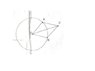# Hard Vector Question Much Help Needed

Karma
Hard Vector Question Much Help Needed!

Points A and B are any two points on a cirlce with a center O. The heads of Vectors OA and OB lie on the circle. Vector OC is the sum of OA and OB . In the Diagram, the head of OC lies outside the circle. Explain your answer to each question.

a.)Is it Possible for the head of OC to lie on the Circle?

b.)Is it Possible for the head of OC to lie inside the circle?

c.)Where are all the possible positions for C?

Here is the Diagram! Thank you any help is appreciated. I tried doing some of the questions but All my answers were wrong, and i got confused, please help me! thank you very much

#### Attachments

•Diagram.JPG
6.3 KB · Views: 616

Homework Helper
I haven't seen the diagram, but I think the notation makes it clear.

a) If the head of OC is on the circle, then it would be the same length as OA and OB. When you add OA and OB graphically, you'll be making a triangle with OC. Is there a triangle in which all three sides are the same length? If so, what can you say about that triangle?

b) If the head is inside the circle, then OC will be shorter than OA and OB (which, if you think about it, must be the same length). Is it possible to have a triangle with two sides the same length and the third side shorter?

c) Picture taking OA and OB and joining the head of OA to the tail of OB. OC will be the third side of the triangle formed. Let the angle between OA and OB vary - what are the possible lengths for the third side?

Note: If the notation isn't quite as clear as I thought it was, I may have to modify these answers when the diagram shows up.

Homework Helper

Karma
a) If the head of OC is on the circle, then it would be the same length as OA and OB. When you add OA and OB graphically, you'll be making a triangle with OC. Is there a triangle in which all three sides are the same length? If so, what can you say about that triangle?

The triangle is an equilateral triangle in which all sides are the same or all angles are 60.. how does this help justify the answer though?

b) If the head is inside the circle, then OC will be shorter than OA and OB (which, if you think about it, must be the same length). Is it possible to have a triangle with two sides the same length and the third side shorter?

Yes it is Possible

c) Picture taking OA and OB and joining the head of OA to the tail of OB. OC will be the third side of the triangle formed. Let the angle between OA and OB vary - what are the possible lengths for the third side?

Sorry about that can you make this a little more clear

Im sorry if i sound stupid or anything, its just that I am taking this course one year up, and normally I am not supposed so I am not as qualified

Homework Helper
You're not stupid, luv. You're just inexperienced in this stuff. That will change.

On the first one, asking about the triangle is just an outgrowth of the mathematician in my soul.

On the third one: Consider two extreme cases. Suppose OA and OB are antiparallel, so they lie along the same line. Next, suppose they're parallel, so again they lie along the same line. What would the difference between the first case and the second case be in terms of the length of the "third" side?

It may help to sketch these things out.